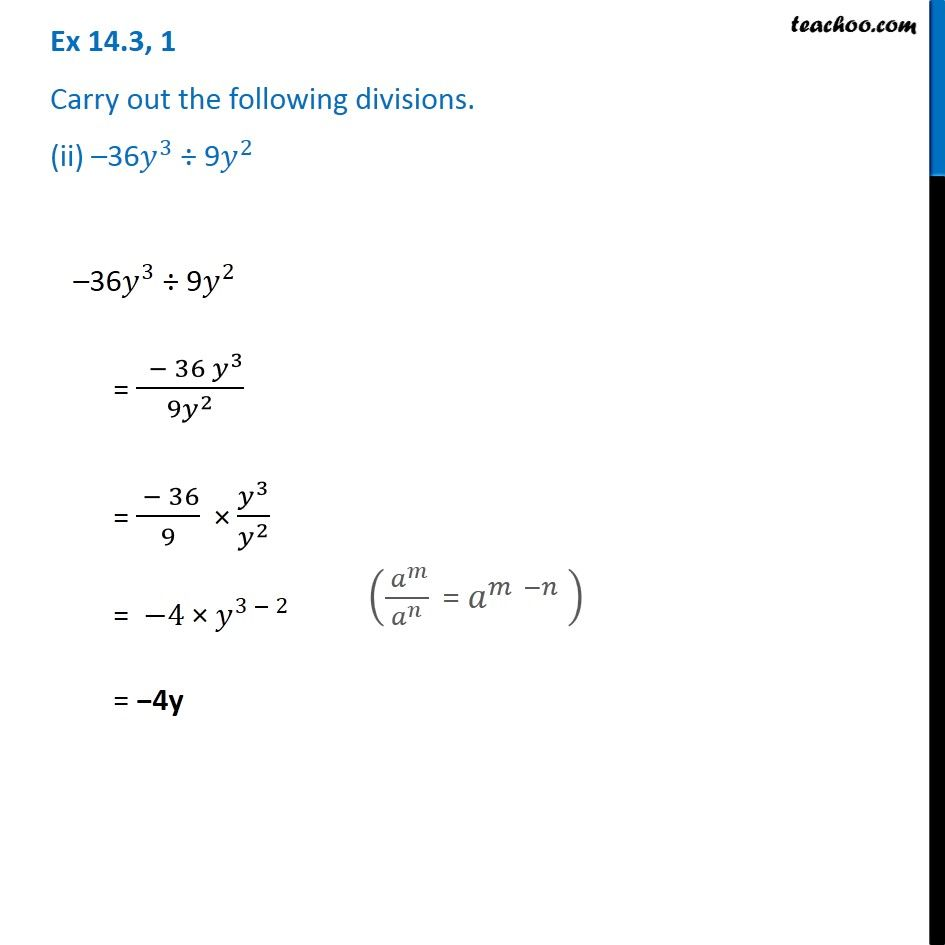1. Chapter 14 Class 8 Factorisation
2. Serial order wise
3. Ex 14.3

Transcript

Ex 14.3, 1 Carry out the following divisions. (ii) –36𝑦^3 ÷ 9𝑦^2 –36𝑦^3 ÷ 9𝑦^2 = ( − 36 𝑦^3)/(9𝑦^2 ) = ( − 36)/9 × 𝑦^3/𝑦^2 = −4 × 𝑦^(3 − 2) = −4y (( 𝑎^𝑚)/𝑎^𝑛 " = " 𝑎^(𝑚 −𝑛) " " )

Ex 14.3